Public marks with tag "math tools" http://blogmarks.net/marks/tag/math tools Create a Graph http://nces.ed.gov/nceskids/Graphing/ Online Graphing tool with ability to download as image 2007-01-20T13:27:57Z knann msvt, district, math, math tools, graphing, ms:math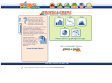#### Create a Graphby knann & 7 others (via)
]]>
Flasks and Graphs http://www.cleo.net.uk/resources/displayframe.php?src=269/consultants_resources%2Fmaths%2Fmaths%2Ffgplusi%2FallFlasks180105.html+ Visual tool for seeing how the filling of various containers graphs over time. 2007-01-20T11:26:27Z knann math tools, msvt, district, projector, ms:mathby knann
Visual tool for seeing how the filling of various containers graphs over time.
]]>
ClassZone - Math Thematics Book 3 http://www.classzone.com/books/math_thematics3/ Web site for Grade 8 math. Includes e-tutorials 2007-01-20T13:25:07Z knann gr8, ms:math, math, math tools, msvt, district, mslc#### ClassZone - Math Thematics Book 3by knann
Web site for Grade 8 math. Includes e-tutorials
]]>
ClassZone - Math Thematics Book 2 http://www.classzone.com/books/math_thematics2/ Web site for Grade 7 math. Includes e-tutorials 2007-01-20T13:25:36Z knann mslc, math tools, gr7, msvt, district, ms:math, msteacher:math#### ClassZone - Math Thematics Book 2by knann
Web site for Grade 7 math. Includes e-tutorials
]]>
ClassZone - Math Thematics Book 1 http://www.classzone.com/books/math_thematics1/ Web site for Grade 6 math. Includes e-tutorials 2007-01-20T13:26:24Z knann ms:math, district, msvt, math tools, gr6, mslc, msteacher:math#### ClassZone - Math Thematics Book 1by knann
Web site for Grade 6 math. Includes e-tutorials
]]>
Mathmatical Toolkit http://lgfl.skoool.co.uk/content.aspx?id=657 In collaboration with Intel and The Mathematical Association, the Mathematical Toolkit and Number line have been designed specifically to support the teaching and learning of mathematics for 2007-01-20T13:54:15Z knann mslc, msteacher:math, math, district, msvt, math tools, teacher resource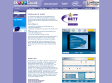#### Mathmatical Toolkitby knann
In collaboration with Intel and The Mathematical Association, the Mathematical Toolkit and Number line have been designed specifically to support the teaching and learning of mathematics for
]]>
Discovering the Value of Pi http://www.arcytech.org/java/pi/index.html The students will measure the diameter and circumference of many circles and will estimate their relationship. By doing so, they should discover the approximate value of pi. By discovering it on their own they should feel rewarded and gain a deeper understanding of the fundamental concept of pi. 2007-01-20T11:31:53Z knann problem solving, geometry, ms:math, district, msvt, math tools, gr8, gr7, gr6#### Discovering the Value of Piby knann
The students will measure the diameter and circumference of many circles and will estimate their relationship. By doing so, they should discover the approximate value of pi. By discovering it on their own they should feel rewarded and gain a deeper understanding of the fundamental concept of pi.
]]>
Math Through Culture:Culturally-Situated Design Tools http://www.rpi.edu/%7Eeglash/csdt.html Many cultural designs are based on mathematical principles. This software will help students learn standards-based mathematics as they simulate the original artifacts, and develop their own creations. 2007-01-20T11:22:50Z knann gr6, gr7, gr8, math tools, msvt, district, math, geometry, pre-algebra, ms:math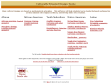#### Math Through Culture:Culturally-Situated Design Toolsby knann (via)
Many cultural designs are based on mathematical principles. This software will help students learn standards-based mathematics as they simulate the original artifacts, and develop their own creations.
]]>
NCTM : Illuminations Lessons http://illuminations.nctm.org/tools/index.aspx Interactive Math tools for grades for K-12. Accompanied by lesson plans and NCTM standards 2007-01-20T11:34:02Z knann gr8, gr3, gr4, gr5, gr9-12, math tools, esvt, gr7, gr6, gr2, gr1, msvt, district, math, es:math, ms:math, hs:math#### NCTM : Illuminations Lessonsby knann
Interactive Math tools for grades for K-12. Accompanied by lesson plans and NCTM standards
]]>
National Library of Virtual Manipulatives http://nlvm.usu.edu/en/nav/vlibrary.html Library of Java Applets aligned to math strands and standards. Many items similar to Investigations software. Click Grade level for specific strand. 2007-01-20T13:56:48Z knann district, esvt, math tools, math, teacher resource, grk-12, math:nlvm, es:math, ms:math, hs:math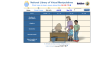#### National Library of Virtual Manipulativesby knann (via)
Library of Java Applets aligned to math strands and standards. Many items similar to Investigations software. Click Grade level for specific strand.
]]>
Math Tools Grade 4 http://mathforum.org/mathtools/cell/m4,ALL,tool,hh/ Use the Math 4 Topics dropdown box to filter the results 2005-10-28T09:33:58Z knann gr4, math tools, esvt, districtby knann
Use the Math 4 Topics dropdown box to filter the results
]]>
Meet the Graphs http://www.teachersfirst.com/lessons/spreadsheets/set1.cfm A brief slide show introducing a spreadsheet and pie/bar graphs. Appropriate for gr4-5 2005-10-28T09:34:52Z knann math, gr4, gr5, math tools, esvt, district, data analysis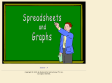#### Meet the Graphsby knann
A brief slide show introducing a spreadsheet and pie/bar graphs. Appropriate for gr4-5
]]>
More Than Math http://www.morethanmath.org/activities.htm More Than Math integrates the visual arts into the 3rd, 4th and 5th grade mathematics curriculum using works of art to explore pattern, symmetry, proportion, perspective, balance and geometric form 2005-12-08T09:35:54Z knann gr3, gr4, gr5, math tools, esvt, district, math, geometry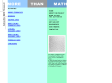#### More Than Mathby knann & 1 other
More Than Math integrates the visual arts into the 3rd, 4th and 5th grade mathematics curriculum using works of art to explore pattern, symmetry, proportion, perspective, balance and geometric form
]]>
Project Interactivate http://www.shodor.org/interactivate/ The goals of Project Interactivate are the creation, collection, evaluation, and dissemination of interactive Java-based courseware for exploration in science and math. 2006-09-25T10:57:30Z knann simulations, lessons, teacher resource, gr6, gr7, gr8, gr9-12, math tools, msvt, hsvt, district, math, hs:math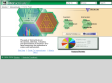#### Project Interactivateby knann
The goals of Project Interactivate are the creation, collection, evaluation, and dissemination of interactive Java-based courseware for exploration in science and math.
]]>